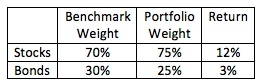### CFA Practice Question

There are 136 practice questions for this study session.

### CFA Practice Question

Continue with the previous question. Consider the following benchmark and managed portfolio.What is the value added for the managed portfolio?

A. 0.45%
B. 0.58%
C. 0.75%

Approach 1:
The return on the managed portfolio: 0.75 x 12% + 0.25 x 3% = 9.75%
The return on the benchmark: 0.70 x 12% + 0.3 x 3% = 9.3%
RA = 9.75% - 9.3% = 0.45%

Approach 2:
RA = ΣΔwiRi = 0.05 x 12% + (-0.05) x 3% = 0.45%

Approach 3:
Portfolio benchmark return = 0.7 x 12% + 0.3 x 3% = 9.3%
RA = ΣΔwiRAi = 0.05 x (12% - 9.3%) - 0.05 x (9.3% - 3%) = 0.45%